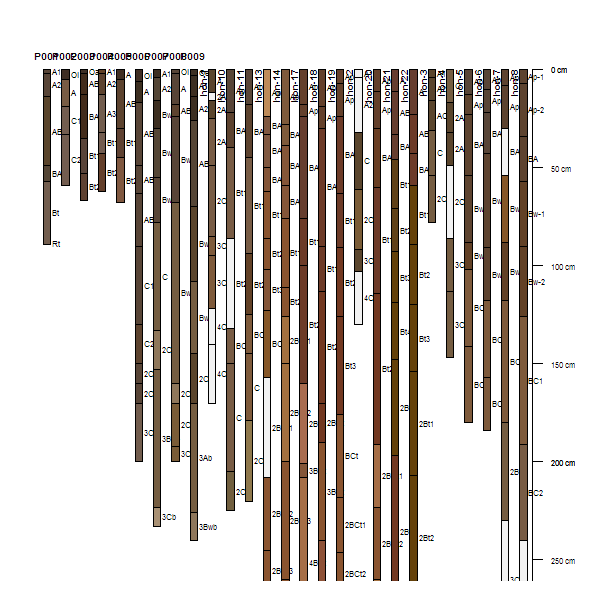SoilProfileCollection-plotting-methods {aqp} R Documentation

## Profile Plot

### Description

Generate a simple diagram of a soil profile, with annotated horizon names.

### Usage

```plotSPC(x, color='soil_color', width=0.2, name=NULL, label=idname(x),
alt.label=NULL, alt.label.col='black', cex.names=0.5,
cex.depth.axis=cex.names, cex.id=cex.names+(0.2*cex.names),
print.id=TRUE, id.style='auto', plot.order=1:length(x), add=FALSE,
scaling.factor=1, y.offset=0, x.idx.offset=0, n=length(x),
max.depth=max(x), n.depth.ticks=5, shrink=FALSE,
shrink.cutoff=3, abbr=FALSE, abbr.cutoff=5, divide.hz=TRUE,
hz.distinctness.offset=NULL, hz.distinctness.offset.col='black',
hz.distinctness.offset.lty=2, axis.line.offset=-2.5,
plot.depth.axis=TRUE, density=NULL, col.label=color,
col.palette = rev(brewer.pal(10, 'Spectral')), lwd=1, lty=1,
default.color=grey(0.95), ...)
```

### Arguments

 `x` a SoilProfileCollection object `color` the name of the column containing R-compatible color descriptions, or a column containing numeric data; see details `width` scaling of profile widths `name` the name of the (horizon-level) attribute containing horizon designation labels `label` the name of the (site-level) attribute used to identify profiles in the plot `alt.label` the name of a (site-level) attribute used for seconary annotation `alt.label.col` color used when printing secondary annotation `cex.names` character scaling applied to horizon names `cex.depth.axis` character scaling applied to depth scale `cex.id` character scaling applied to profile id `print.id` should the profile id be printed above each profile? (TRUE) `id.style` profile ID printing style: 'auto' (default) = simple heuristic used to select from: 'top' = centered above each profile, 'side' = 'along the top-left edge of profiles' `plot.order` a vector describing the order in which individual SoilProfile objects from the parent should be plotted `add` add to an existing figure `scaling.factor` vertical scaling of the profile heights `y.offset` vertical offset for top of profiles `x.idx.offset` integer specifying horizontal offset from 0 `n` integer describing amount of space along x-axis to allocate, defaults to length(x) `max.depth` suggested lower depth boundary of plot `n.depth.ticks` suggested number of ticks in depth scale `shrink` should long horizon names be shrunk by 80% ? `shrink.cutoff` character length defining long horizon names `abbr` should the profile ID be abbreviated? `abbr.cutoff` suggested minimum length for abbreviated IDs `divide.hz` should horizons be divided with a thin black line? (default is TRUE) `hz.distinctness.offset` column name containing vertical offsets used to depict horizon boundary distinctness (same units as profiles) `hz.distinctness.offset.col` color used to encode horizon distinctness (default is 'black') `hz.distinctness.offset.lty` line style used to encode horizon distinctness (default is 2) `axis.line.offset` horizonatal offset applied to depth axis (default is -2.5) `plot.depth.axis` plot depth axis? (default is TRUE) `density` fill density used for horizon color shading, either a single integer or a column name containing integer values (default is NULL, no shading) `col.label` text printed above the color-coded legend `col.palette` color palette used to plot numeric data `lwd` single numeric value: line width multiplier `lty` single integert: line style `default.color` default horizon fill color used when 'color' attribute is NA `...` other arguments passed into lower level plotting functions

### Details

Depth limits (`max.depth`) and number of depth ticks (`n.depth.ticks`) are *suggestions* to the `pretty` function. You may have to tinker with both parameters to get what you want.

The 'side' `id.style` is useful when plotting a large collection of profiles, and/or, when profile IDs are long.

If the column containing horizon designations is not specified (the `name` argument), a column (presumed to contain horizon designation labels) is guessed based on regular expression matching of the pattern 'name'– this usually works, but it is best to manual specify the name of the column containing horizon designations.

The `color` argument can either name a column containing R-compatible colors, possibly created via `munsell2rgb`, or column containing numeric values. In the second case, numeric values are converted into colors and displayed along with a simple legend above the plot. Note that this functionality makes several assumptions about plot geometry and is most useful in an interactive setting.

The `x.idx.offset` argument can be used to shift a collection of pedons from left to right in the figure. This can be useful when plotting several different `SoilProfileCollection` objects within the same figure. Space must be pre-allocated in the first plotting call, with an offset specified in the second call. See examples below.

### Value

A new plot of soil profiles is generated, or optionally added to an existing plot.

### Methods

`signature(x = "SoilProfileCollection")`

### Author(s)

Dylan E. Beaudette

### References

http://casoilresource.lawr.ucdavis.edu/

`SoilProfileCollection-class, pretty, hzDistinctnessCodeToOffset, addBracket, profileGroupLabels`

### Examples

``````data(sp1)

# usually best to adjust margins
par(mar=c(0,0,3,0))

# add color vector
sp1\$soil_color <- with(sp1, munsell2rgb(hue, value, chroma))

# promote to SoilProfileCollection
depths(sp1) <- id ~ top + bottom

# plot profiles
plot(sp1, id.style='side')

# title, note line argument:
title('Sample Data 1', line=1, cex.main=0.75)
``````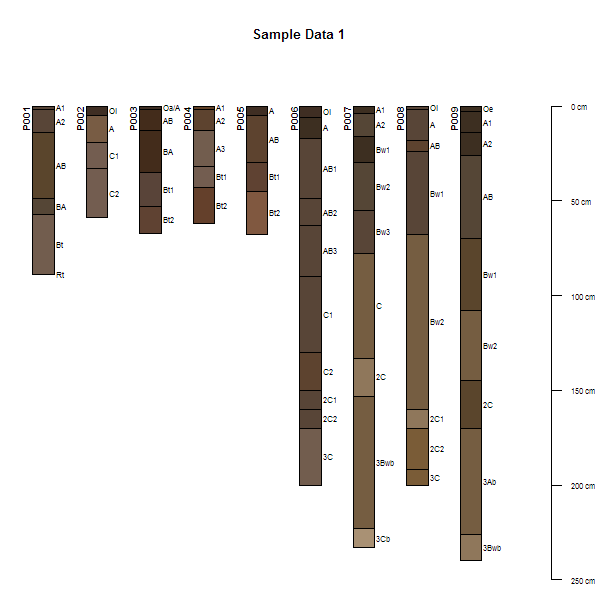``````# plot profiles without horizon-line divisions
plot(sp1, divide.hz=FALSE)
``````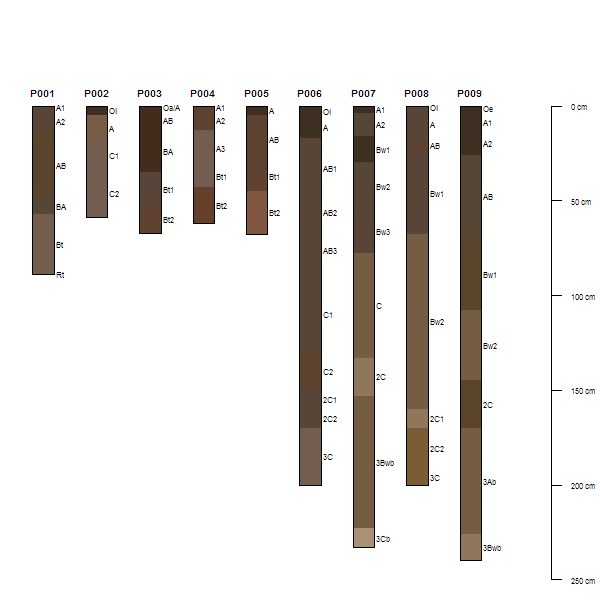``````# add dashed lines illustrating horizon boundary distinctness
sp1\$hzD <- hzDistinctnessCodeToOffset(sp1\$bound_distinct)
plot(sp1, hz.distinctness.offset='hzD')
``````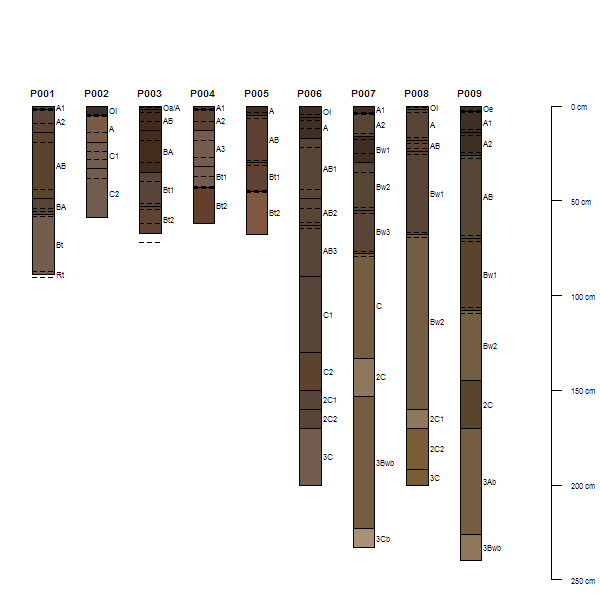``````# plot horizon color according to some property
data(sp4)
depths(sp4) <- id ~ top + bottom
plot(sp4, color='clay')
``````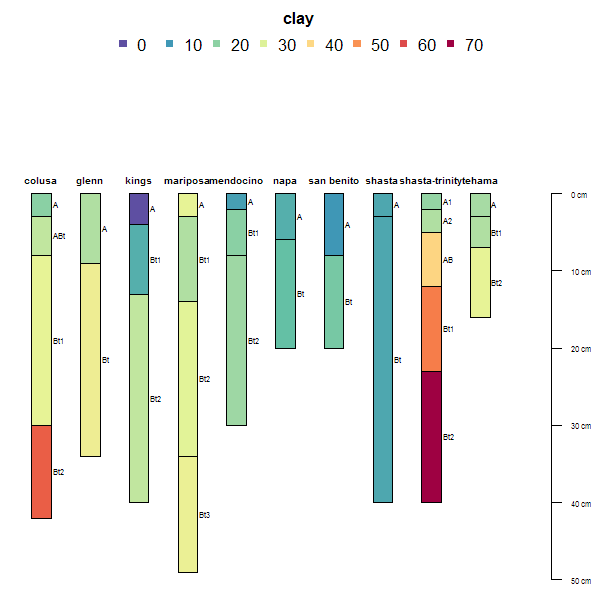``````# another example
data(sp2)
depths(sp2) <- id ~ top + bottom
site(sp2) <- ~ surface

# label with site-level attribute: `surface`
plot(sp2, label='surface', plot.order=order(sp2\$surface))
``````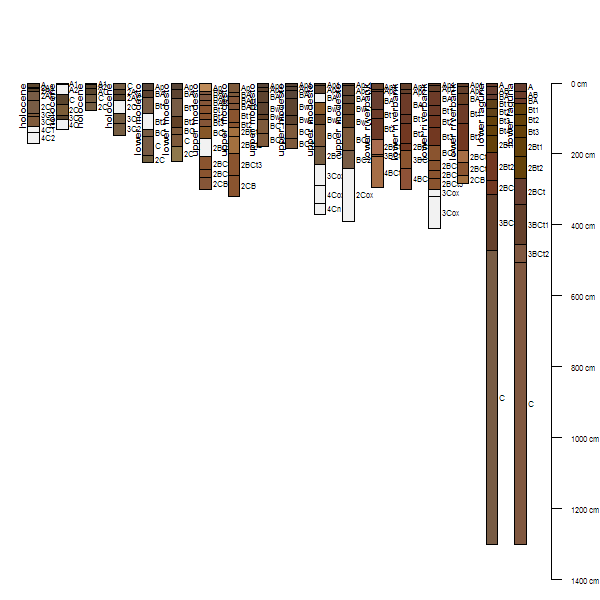``````# plot two SPC objects in the same figure
par(mar=c(1,1,1,1))
# plot the first SPC object and
# allocate space for the second SPC object
plot(sp1, n=length(sp1) + length(sp2))
# plot the second SPC, starting from the first empty space